Graphics Output

This section describes the use of ODS for creating graphics with the SEQDESIGN procedure. To request these graphs, ODS Graphics must be enabled and you must specify the associated graphics options in the PROC SEQDESIGN statement. Except for the PLOTS=BOUNDARY option, where a detailed boundary plot is generated for each design separately, each option produces a plot for all designs together. For more information about ODS Graphics, see Chapter 21, Statistical Graphics Using ODS.

### Sequential ASN Plot

The PLOTS=ASN option displays the average sample numbers (expected sample sizes for nonsurvival data or expected numbers of events for survival data) under various hypothetical references. The average sample numbers are connected for each design, and these connected curves for all designs are displayed in the "Sequential ASN Plot" graph.

For a one-sided design, average sample numbers under the hypothetical references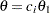are displayed, where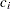are the values specified in the CREF= option and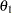is the alternative reference. The horizontal axis displays thevalues of these hypothetical references.

For a two-sided design, average sample numbers under each of the hypothetical referencesand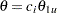are displayed, where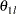and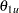are the lower and upper alternative references, respectively. The horizontal axis displays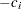values for lower hypothetical referencesandvalues for upper hypothetical references.

Note that for a symmetric two-sided design, only average sample numbers under the hypothetical referencesare derived.

### Sequential Boundary Plot

The PLOTS=BOUNDARY option displays boundary values and the acceptance and rejection regions at each stage for each design separately in the "Detailed Boundary Information" graph. The BOUNDARYSCALE= option is used to specify the scale of the boundaries on the vertical axis. The keywords MLE, SCORE, STDZ, and PVALUE in the BOUNDARYSCALE= option correspond to the boundary with the MLE scale, score statistic scale, standardized normal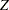scale, and-value scale, respectively.

The stage numbers are displayed on the horizontal axis. In addition, the HSCALE= option in the PLOTS=BOUNDARY option can be used to specify the scale on the horizontal axis. The keywords INFO and SAMPLESIZE in the HSCALE= option correspond to the information levels and sample sizes, respectively.

### Combined Sequential Boundary Plot

The PLOTS=COMBINEDBOUNDARY option displays boundary values. The boundary values are connected for each boundary in each design, and these connected curves for all designs are displayed in the "Sequential Boundary Information" graph. The BOUNDARYSCALE= option is used to specify the scale of the boundaries on the vertical axis. The keywords MLE, SCORE, STDZ, and PVALUE in the BOUNDARYSCALE= option correspond to the boundary with the MLE scale, score statistic scale, standardized normalscale, and-value scale, respectively.

The HSCALE= option in the PLOTS=COMBINEDBOUNDARY option can be used to specify the scale on the horizontal axis. The keywords INFO, SAMPLESIZE, and STAGE in the HSCALE= option correspond to the information levels, sample sizes, and stage numbers, respectively.

### Sequential Error Spending Plot

The PLOTS=ERRSPEND option displays the cumulative error spending at each stage on each boundary in the "Sequential Error Spending Plot" graph. A legend table uses the design labels to identify the curves for the corresponding design in the plot. Another legend table uses symbols to identify boundaries in the plot.

### Sequential Power Plot

The PLOTS=POWER option displays the powers under various hypothetical references. The powers are connected for each design, and these connected curves for all designs are displayed in the "Sequential Power Plot" graph.

For a one-sided design, powers under hypothetical referencesare displayed, whereare the values specified in the CREF= option andis the alternative reference. The horizontal axis displays thevalues of these hypothetical references.

For a two-sided design, powers under hypothetical referencesandare displayed, whereandare the lower and upper alternative references, respectively. The horizontal axis displaysvalues for lower hypothetical referencesandvalues for upper hypothetical references.

Note that for a symmetric two-sided design, only powers under hypothetical referencesare derived.